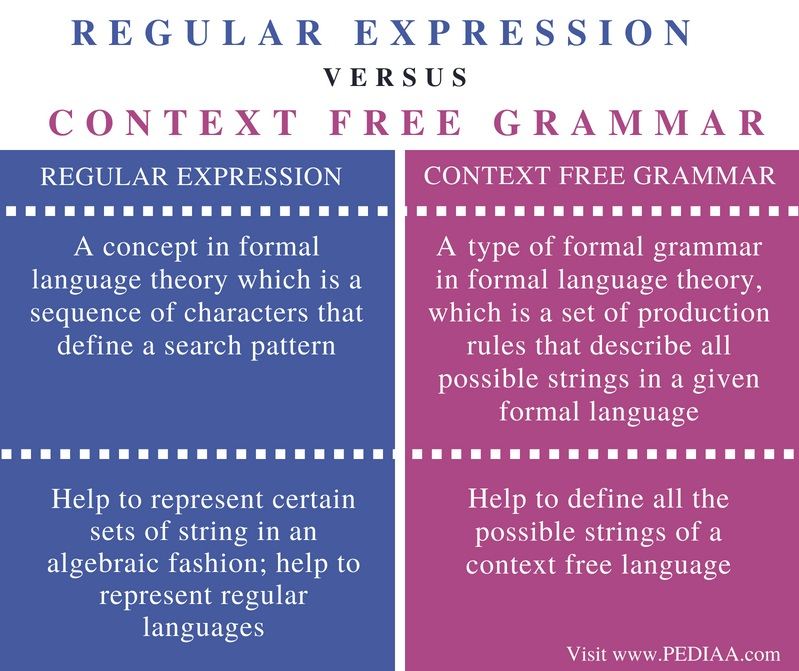# Difference Between Regular Expression and Context Free Grammar

The main difference between regular expression and context free grammar is that the regular expressions help to describe all the strings of a regular language while the context free grammar helps to define all possible strings of a context free language.

Grammar denotes syntactical rules for conversation in natural languages. Computer Science uses the theory of formal languages to a great extent. In the year 1956, Noam Chomsky gave a mathematical model of grammar for writing computer languages. When it is possible to derive a set of all strings from a grammar, it is said that the language is generated from that grammar. Two types of grammar are regular grammar and context free grammar. Any language that can be described by a regular expression is a regular language. Context free grammar is a generalization of regular expression. It is possible to use regular expressions to write regular languages and context free grammar to write context free grammar.

### Key Areas Covered

1. What is Regular Expression
– Definition, Examples
2. What is Context Free Grammar
– Definition, Examples
3. Relationship Between Regular Expression and Context Free Grammar
– Outline of Association
4. Difference Between Regular Expression and Context Free Grammar
– Comparison of Key Differences

### Key Terms

Regular Expression, Context Free Grammar## What is Regular Expression

The regular grammar generates regular languages. This grammar has a single non-terminal on the left-hand side and a right-hand side consisting of a single terminal or single terminal followed by a single non-terminal. It can have a production rule as follows.

X -> a or X -> a Y

Where X, Y ϵ N (non-terminal) and a ϵ T (terminal)

Regular expressions help to write regular grammar to describe regular languages.A regular expression represents a certain set of strings in an algebraic fashion. Some important rules to follow when writing a regular expression are as follows.

1. The terminal symbols, null symbol and empty symbol are regular expressions.
2. The union of two regular expressions is a regular expression.
3. The concatenation of two regular expressions is a regular expression.
4. Iteration or closure is a regular expression.

The regular expression for the set {0,1,2} is as follows.

R = 0 + 1+2

The set {abb,a,b, bba} can be represented by the following regular expression.

R = abb + a +b + bba

Consider the set, {ϵ, 0, 00, 000, …}

The ϵ is the empty string. The regular Expression is R = 0*. This represents the closure of the symbol including the empty symbol.

In the set {1, 11, 111, 1111,  …..}

The regular expression is R = 1 +.  This + denotes the closure of a symbol excluding the empty symbol.

## What is Context Free Grammar

In formal language theory, Context Free Language (CFL) is a language generated by Context Free Grammar. Four parameters define context free grammar (G).

G= {V, ∑ , S, P}

V: Set of Variable or Non Terminal Symbols.

∑: Set of terminal symbols

S: Start Symbol

P: Production Rule

Context Free Grammar has the following format for production rule.

A -> a  where  a = {V, ∑ }* and  A ϵ V

One example of Context Free Grammar is as follows. Each production consists of a non-terminal symbol and a regular expression.

For generating a language that generates an equal number of a’s and b’s is in the format of anbn. The context free grammar is as follows.

G = {(S,A), (a,b), (S ->aAb, A -> aAb | ϵ )}

Considering the start symbol,

S – > a A b

By applying A -> aAb

→ a a  A b b

By applying  A -> aAb again,

→ a a a A b b b

By applying A -> ϵ  (This symbol denotes an empty string)

→ a a a b b b

→ a 3 b 3

When considering the output, the number of a’s is equal to number of b’s. It has the  an bn form.

## Relationship Between Regular Expression and Context Free Grammar

• Context free grammar is a generalization of regular expressions.

## Difference Between Regular Expression and Context Free Grammar

### Definition

A regular expression is a concept in formal language theory which is a sequence of characters that define a search pattern. Context Free Grammar is a type of formal grammar in formal language theory, which is a set of production rules that describe all possible strings in a given formal language.

### Usage

Regular expressions help to represent certain sets of string in an algebraic fashion. It helps to represent regular languages. Context free grammar helps to define all the possible strings of a context free language.

### Conclusion

A regular expression is a method for pattern matching. It is a flexible method of providing a flexible and concise means to match strings of text. It defines all strings in the regular language. On the other hand, context free grammar allows defining all the strings belonging to a context free language. The difference between regular expression and context free grammar is that the regular expressions help to describe all the strings of a regular language while the context free grammar helps to define all possible strings of a context free language.

##### Reference:

1. “Regular Expressions.” Www.tutorialspoint.com, Tutorials Point, 8 Jan. 2018, Available here.
2. “Context-Free Grammar Introduction.” Www.tutorialspoint.com, Tutorials Point, 8 Jan. 2018, Available here.

##### Image Courtesy:

1. “Toolbaricon RegEx” By M0tty – Own work (CC BY-SA 4.0) via Commons Wikimedia